output.to from Sideway
Mechanics: StaticsMechanics Dynamics

Friction ForceExamples of Friction ForceApplications of Friction

Draft for Information Only

# Content

``` Examples of Friction Forces  Sliding Block on inclined board  Ladder on Wall Problem  Ladder on Edge Problem```

# Examples of Friction Forces

Frictional forces can be found in common practical engineering problems.

## Sliding Block on inclined board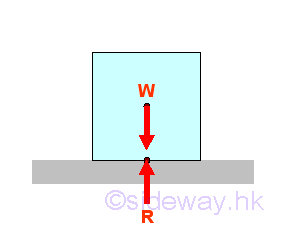When a sliding block is placed on a horizontal board without subject to other force, there are only the weight W and the reaction R. The reaction force R balances the weight W. Since there is no horizontal force component acts on the contact surfaces, the friction force between the surfaces of contact is zero also.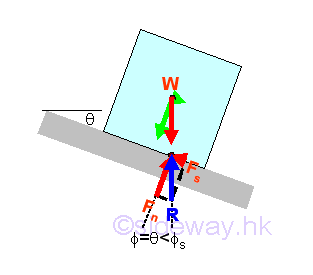When increasing the inclination of the board by a small angle θ, the reaction force R which balances the weight W, will deviate from the normal of the board surface by the angle θ also. The reaction force R can be converted into normal component Fn of magnitude W cos θ and tangential component Fs  of magnitude W sin θ with respect to the board surface through force discomposition. If the magnitude of the tangential component of the weight W  is less than the maximum static friction force Fs,max , the reactive friction force Fs is equal to the W sin θ also. And the angle of inclination θ of the board is equal to the angle of friction but  the angle of friction is smaller than the angle of static friction.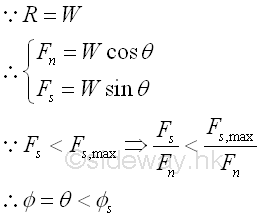When the motion of solid body is impending.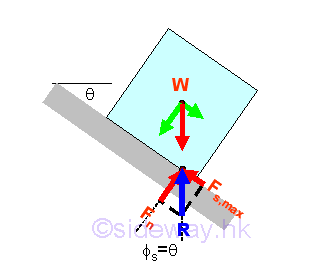When increasing the angle of inclination θ, the tangential component of the weight along the board surface will increase also. This tangential force which causes the sliding down motion of the block, should be balanced by the static friction force. When the angle of inclination θ is increased to cause the solid body to just about to move, the angle of inclination is called the angle of repose. Since the block is in static equilibrium, the reaction R is equal to the weight W. Similarly, the reaction force R can be converted into normal component Fn of magnitude W cos θ and tangential component Fs,max  of magnitude W sin θ with respect to the board surface through force discomposition. If the magnitude of the tangential component of the weight W  is balanced by the maximum static friction force Fs,max , the reactive friction force Fs,max is equal to the W sin θ also. And the angle of inclination θ of the board is equal to the angle of static friction øs also. imply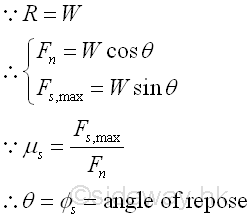When the solid body is sliding.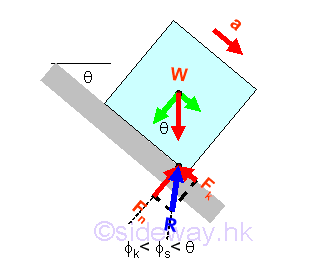The further increasing of the angle of inclination θ, the tangential component of the weight along the board surface will further increase also. Since the further increased tangential force due to the weight cannot be balanced by the maximum static friction force any more, the incresed tangential force will cause the block to move and to slide down. Because the maximum static friction force is smaller than the tangential component of the weight, the resultant reaction force R is smaller than the weight W. Similarly, when the block is sliding, the reaction force R can be converted into normal component Fn of magnitude W cos θ and tangential component Fk of magnitude μkFn with respect to the board surface through force discomposition. Since the normal component of reaction Fn is equal to the normal component of weight W, and the tangential component of reaction Fk is smaller than the tangential component of weight W, the angle of kinetic friction øs is smaller than the angle of inclination θ also. Imply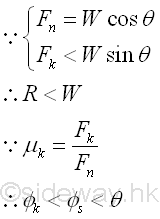Therefore by increasing the angle of inclination, the maximum possible position of the point of application of the weight W  on the contact surfaces is at the corner of block. Imply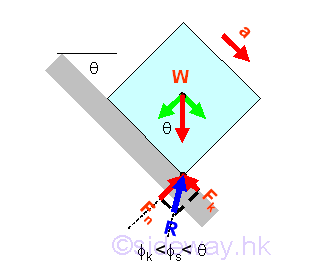If the magnitude of the tangential component of the weight W is less than the maximum value of the static friction force Fs,max when the point of application shifting to the corner of the block, the further increasing the magnitude of the anlge of inclination will tip over the block about the corner of the block because of the formed resultant couple WF.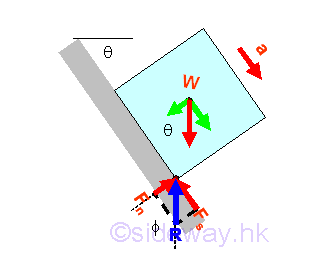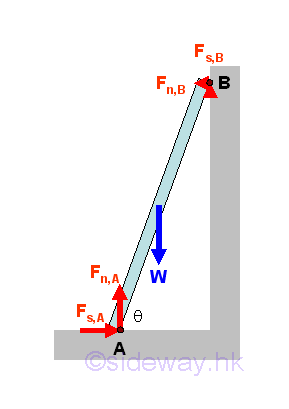A ladder leans against a wall at angle θ with friction of identical friction coefficients μ acts at both A and B. The minimum value of friction coefficient for the ladder such that the ladder will stand without slipping can be determined by equilibrium equations. Imply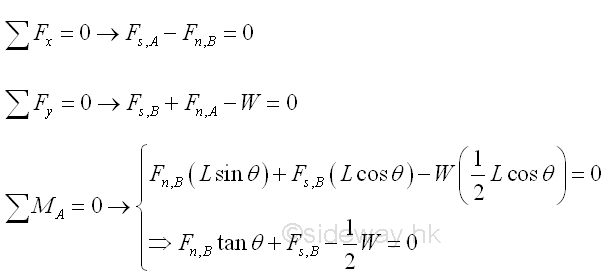Besides, for friction of identical friction coefficeints, imply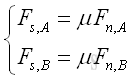The minimum value of friction coefficient is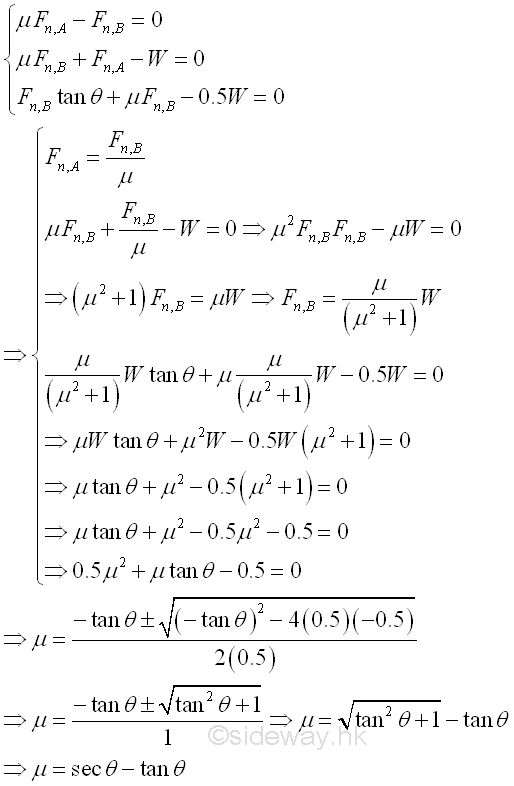Therefore, the reaction forces of the ladder are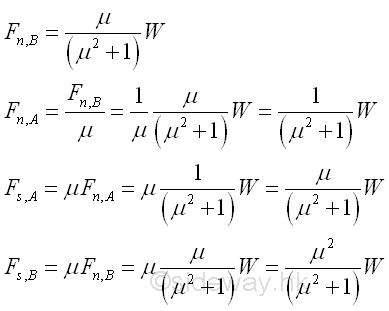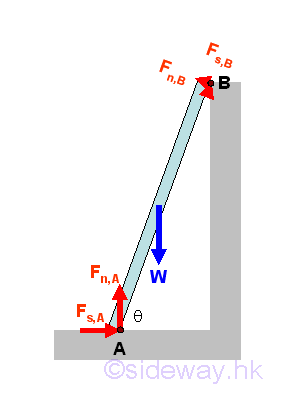A ladder leans against an edge at angle θ with friction of identical friction coefficients μ acts at both A and B. The minimum value of friction coefficient for the ladder such that the ladder will stand without slipping can be determined by equilibrium equations. Imply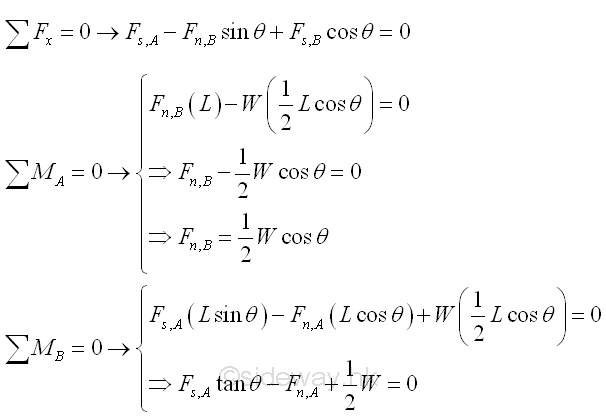Besides, for friction of identical friction coefficeints, implyThe minimum value of friction coefficient is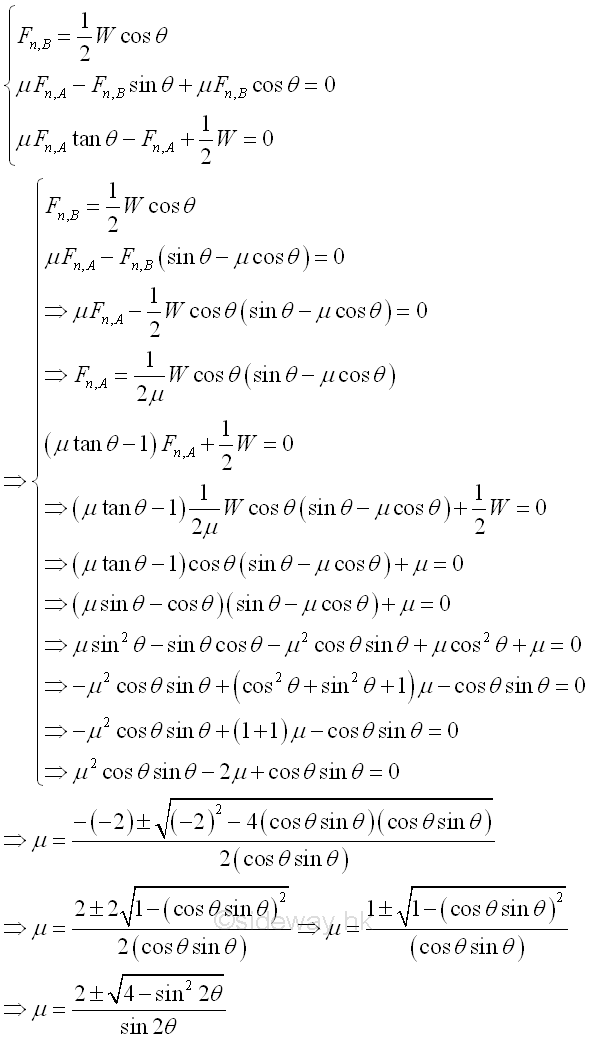Therefore, the reaction forces of the ladder are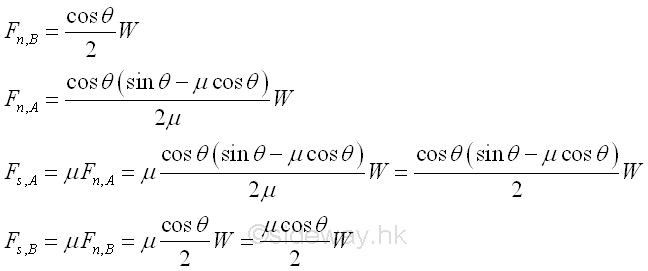ID: 120800001 Last Updated: 8/2/2012 Revision: 0 Ref:References

1. I.C. Jong; B.G. rogers, 1991, Engineering Mechanics: Statics and Dynamics
2. F.P. Beer; E.R. Johnston,Jr.; E.R. Eisenberg, 2004, Vector Mechanics for Engineers: StaticsHome 5

Management

HBR 3

Information

Recreation

Culture

Chinese 1097

English 339

Computer

Hardware 226

Software

Application 213

Latex 52

Manim 205

KB 1

Numeric 19

Programming

Web 289

Unicode 504

HTML 66

CSS 65

SVG 46

ASP.NET 270

OS 429

Python 72

Knowledge

Mathematics

Algebra 84

Geometry 34

Calculus 67

Engineering

Mechanical

Rigid Bodies

Statics 92

Dynamics 37

Control

Natural Sciences

Electric 27